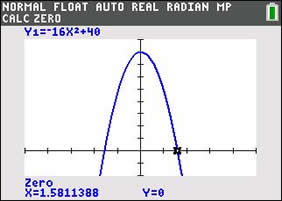Projectile (Vertical) Motion Terms of Use    Contact Person: Donna RobertsA projectile is an object that rises and falls under the influence of gravity, and projectile motion is the height of that object as a function of time. Projectile motion can be modeled by a quadratic function. (Air resistance will be ignored in these problems.)

Projectile motion involves objects that are dropped, thrown straight up, or thrown straight down. Factors that influence the height of these object include the height from which the objects are dropped or thrown, whether upward/downward velocity is involved, and of course, the pull of gravity downward on the object. On Earth, the acceleration due to gravity is approximately 32 feet/second2 (or 9.8 meters/second2).

 General Formula: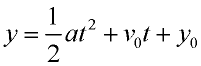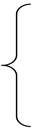y = height t = time in seconds a = acceleration due to gravity v0 = initial (starting) velocity y0 = initial (starting) height
FYI: This formula uses ½ the acceleration since acceleration increases over one second, so the average, or ½a, is used.
Notice that this formula is a quadratic function, which means its graph will be a parabola.Objects that are dropped: If an object is dropped, it is simply released, and not thrown. There is no initial velocity other than the pull of gravity. This means that the middle term of the formula (seen above) is not needed, since initial velocity (v0) = 0. We will now be using "h" (instead of "y") to represent the height (as it is easier to remember its meaning) and h0 to represent the initial (starting) height.

 Working in FEET: The acceleration due to gravity is -32 ft/sec/sec. (gravity pulls objects toward the Earth making the value negative). The formula to model the height of an object t seconds after it has been dropped is: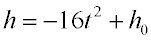Working in METERS: The acceleration due to gravity is -9.8 meters/sec/sec. The formula to model the height of an object t seconds after it has been dropped is: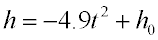Objects that are thrown: If an object is thrown, a certain amount of initial velocity accompanies its launch. Now, we have a reason to use that middle term from the General Formula (seen above). We will continue to use "h" to represent the height and h0 to represent the initial (starting) height.

 Working in FEET: The acceleration due to gravity is -32 ft/sec/sec. The formula to model the height of an object t seconds after it has been thrown is: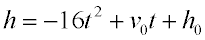Working in METERS: The acceleration due to gravity is -9.8 meters/sec/sec. The formula to model the height of an object t seconds after it has been thrown is: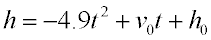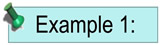A model rocket is launched straight upward. The solid fuel propellant pushes the rocket off the ground at an initial velocity of 200 feet per second. a. When will the rocket reach a height of 336 feet? b. What is the maximum height reached by the rocket? c. You forgot to put the parachute in the rocket. When will the rocket hit the ground?Solution: Choose the formula h = -16t2 + v0t + h0. The initial velocity, v0 = 200 ft/sec and the initial height is h0 = 0 (since it is launched from the ground).
Formula: h = -16t2 + 200t + 0.

 a. Replace h with 336 and solve. 336 = -16t2 + 200t 16t2 - 200t + 336 = 0 8(2t2- 25t + 42) = 0 8 (2t - 21)(t - 2) = 0 2t - 21 = 0; 2t = 21; t = 10.5 sec. t - 2 = 0; t = 2 sec. The rocket reaches a height of 336 feet on its way up after 2 seconds and on its way down after 10.5 seconds.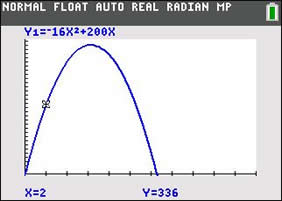b. Maximum height? A parabola reaches its maximum value at its vertex, or turning point. Use the formula for the axis of symmetry to find the x-coordinate of the vertex.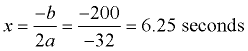Plug in for t and find h. h = -16(6.25)2 + 200(6.25) = 625 ft. The maximum height reached is 625 feet.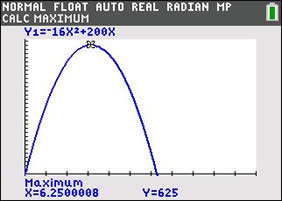c. Hit the ground? The ground will be a height of 0. Set the equation equal to 0. -16t2 + 200t = 0 -8t(2t - 25) = 0 -8t = 0; t = 0 (at launch) 2t - 25 = 0; t = 12.5 sec. The rocket hit the ground 12.5 seconds after launch. (graph at the right)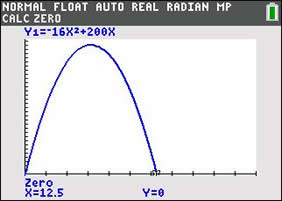Be careful! It may look like the graph is showing the physical "path" of the rocket, but it is not. This graph is showing the rocket's height at specific moments in time (the x-axis is time), as the rocket travels straight up and then straight down.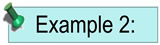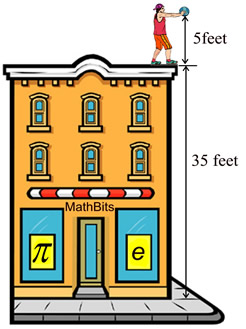Carmine drops a ball at shoulder height from the top of a building (as seen at the left). If the ball is at rest, and is simply dropped, how long will it take, to the nearest tenth of a second, to hit the ground?
Solution:
h = -16t2 + h0    The initial height is 40 feet and the height when the ball hits the ground will be 0.
 0 = -16t2 + 40 16t2 = 40 t2 = 40/16 = 5/2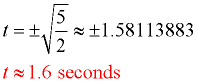We don't consider "negative" time, so there is only one answer.